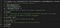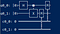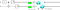# A Quantum Circuit to Model The Application of Entanglement in Quantum computing

Information can be identified as the most general thing which must propagate from a cause to an effect. It therefore has a fundamentally important role in the science of physics. This has meant that the full significance of information as a basic concept in physics is only now being discovered. This is especially true in quantum mechanics. The theory of quantum information and computing puts this significance on a firm footing, and has lead to some profound and exciting new insights into the natural world. Among these are the use of quantum states to permit the secure transmission of classical information (quantum cryptography), the use of quantum entanglement to permit reliable transmission of quantum states (teleportation) etc., This paper will focus on the use of quantum entanglement as a computational resource.

Introduction

The subject of quantum computing brings together ideas from classical information theory, computer science, and quantum physics. The power of quantum computing is based on several phenomena and laws of the quantum world that are fundamentally different from those one encounters in classical computing, complex probability amplitudes, quantum interference, quantum parallelism, quantum entanglement, and the unitarity of quantum evolution. In order to understand these features and to make a use of them for the design of quantum algorithms networks and processors one has to understand several basic principles which quantum mechanics is based on as well as the basics of Hilbert space formalism that represents the mathematical framework used in quantum mechanics. The key features of an ordinary computer — bits, registers, logic gates, algorithms, and so on — have analogous features in a quantum computer. Instead of bits, a quantum computer has quantum bits or qubits, which work in a particularly intriguing way. Where a bit can store either a zero or a 1, a qubit can store a zero, a one, both zero and one, or an infinite number of values in between — and be in multiple states (store multiple values) at the same time.Qubits use superposition to represent multiple states (multiple numeric values) simultaneously in a similar way.

Representation and Measurement of Quantum bit (Qubit)

The state of a single qubit can be described by a two-dimensional column vector of unit norm, that is, the magnitude squared of its entries must sum to 1. This vector, called the quantum state vector, holds all the information needed to describe the one-qubit quantum system just as a single bit holds all of the information needed to describe the state of a binary variable. Any two-dimensional column vector of real or complex numbers with norm 1 represents a possible quantum state held by a qubit. Thus

represents a qubit state if are complex numbers satisfying

. Some examples of valid quantum state vectors representing qubits include

.

Now that we know how to represent a qubit, we can gain some intuition for what these states represent by discussing the concept of measurement. A measurement corresponds to the informal idea of “looking” at a qubit, which immediately collapses the quantum state to one of the two classical states

. When a qubit given by the quantum state vector

is measured, we obtain the outcome 0 with probability

and the outcome 1 with probability

. On outcome 0, the qubit’s new state is

; on outcome 1 its state is

. Note that these probabilities sum up to 1 because of the normalization condition

.

Quantum Superposition and Entanglement

One of the properties that sets a qubit apart from a classical bit is that it can be in superposition. Superposition is one of the fundamental principles of quantum mechanics. In classical physics, a wave describing a musical tone can be seen as several waves with different frequencies that are added together, superposed. Similarly, a quantum state in superposition can be seen as a linear combination of other distinct quantum states. A quantum computer consisting of n qubits can exist in a superposition of

states: from ∣000…0⟩ to ∣111…1⟩. In contrast, playing n musical sounds with all different frequencies, can only give a superposition of n frequencies. Adding classical waves scales linear, where the superposition of quantum states is exponential.

The other counter-intuitive phenomena in quantum physics is entanglement. For example, two particles are created in such a way that the total spin of the system is zero. If the spin of one of the particles is measured on a certain axis and found to be counterclockwise, then it is guaranteed that a measurement of the spin of the other particle (along the same axis) will show the spin to be clockwise. This seems strange, because it appears that one of the entangled particles “feels” that a measurement is performed on the other entangled particle and “knows” what the outcome should be, but this is not the case. This happens, without any information exchange between the entangled particles. They could even be billions of miles away from each other and this entanglement would still be present.antum state of each particle cannot be described independently of the quantum state of the other particle(s). The quantum state of the system as a whole can be described; it is in a definite state, although the parts of the system are not.

Quantum gates

There are lots of gates used in Quantum computing, but for the purpose of this paper I will only be defining two.

Controlled-NOT

The controlled-NOT gate (also C-NOT or CNOT) is a quantum gate that is an essential component in the construction of a quantum computer. It can be used to entangle and disentangle EPR states. Any quantum circuit can be simulated to an arbitrary degree of accuracy using a combination of CNOT gates and single qubit rotations. The CNOT gate operates on a quantum register consisting of 2 qubits. The CNOT gate flips the second qubit (the target qubit) if and only if the first qubit (the control qubit) is

. The symbol of CNOT is

.and the matrix representation is

.

The Hadamard gate is a single-qubit operation that maps the basis state

to

and

, thus creating an equal superposition of the two basis states. The symbol of CNOT is

and the matrix representation is

Quantum Circuit Implementation

You can easily create an EPR pair by means of a circuit consisting of a Hadamard gate on the first qubit, followed by a CNOT gate, having two

states as an input. The calculations would be:

Given 2 qubits of

states, one can easily create an EPR pair by:

1. Hadamard gate acts on the first qubit. This produces the superposition state seen below:

These Bell states tell us that there is an equal probability of a pair of entangled qubits either being in the first configuration or the second configuration in any one of the given four Bell states. For example, in the first Bell state Φ+ the qubits have an equal chance of being either in the |00⟩ or |11⟩ configuration, while in the third Bell state Ψ+ the qubits have an equal chance of being either in the |01⟩ or |10⟩ configuration.

1. To create entanglement between those 2 qubits, we will apply a CNOT gate , making the first qubit the control qubit and the second qubit the target qubit. Recall that CNOT gate flips only the target qubit if the control qubit is in state

Visualization

The diagrams below show the effect of combining the Hadamard operator and the CNOT gate to generate the first Bell state Φ+ given both qubits start in the |0⟩ initial state. If we wanted to generate any of the other Bell states, then we would simply change the initial states of the qubits. The code fragment below shows us creating our initial qubits and adding our operators (gates) our qubits.Implementing quantum circuit code using QISKIT(IBM)The result from the above codeUsing quirk simulator to visualize how hadamard and CNOT gate cause entanglement in quantum circuit. Note that this is another way of visualizing your circuit if you are not interested in coding.

Conclusion and Physical Interpretation

There are many usefulness of Quantum Computer over classical computers. Aside the fact that it can solve large amount of data quickly, quantum computers will be able to read secret messages communicated over the internet using the current technologies (such as RSA, and other cryptographic protocols). I think there are many more applications of quantum computers that are yet to be discovered. You can also join the community of curios quantum scientist and engineers by asking yourself this question: “How will quantum computers be useful?”

QuantumWriter. “Quantum Computing Concepts.” Quantum Computing Concepts | Microsoft Docs, docs.microsoft.com/en-us/quantum/concepts/?view=qsharp-preview.

“Main Page.” Wikipedia, Wikimedia Foundation, 24 Mar. 2019, en.wikipedia.org/wiki?curid=3010688.

“Quantum Computing: A Simple Introduction.” Explain That Stuff, 26 Mar. 2019, www.explainthatstuff.com/quantum-computing.html.

Written by